## How to Calculate and Solve for Isentropic Expansion | Enthalpy

The isentropic expansion is illustrated by the image below.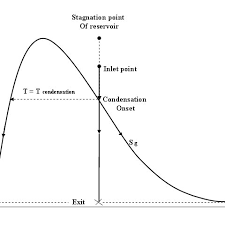To compute for isentropic expansion, two essential parameters are needed and these parameters are Internal Energy 1 (U1) and Internal Energy 2 (U2).

The formula for calculating isentropic expansion:

W = u1 – u2

Where:

W = Isentropic Expansion
u1 = Internal Energy 1
u2 = Internal Energy 2

Given an example;
Find the isentropic expansion when the internal energy 1 is 14 and the internal energy 2 is 8.

This implies that;

u1 = Internal Energy 1 = 14
u2 = Internal Energy 2 = 8

W = u1 – u2
W = 14 – 8
W = 6

Therefore, the isentropic expansion is 6.

Calculating the Internal Energy 1 when the Isentropic Expansion and the Internal Energy 2 is Given.

u1 = W + u2

Where:

u1 = Internal Energy 1
W = Isentropic Expansion
u2 = Internal Energy 2

Let’s solve an example;
Given that the isentropic expansion is 21 and the internal energy 2 is 4. Find the Internal energy 1?

This implies that;

W = Isentropic Expansion = 21
u2 = Internal Energy 2 = 4

u1 = W + u2
u1 = 21 + 4
u1 = 25

Therefore, the internal energy 1 is 25.

## How to Calculate and Solve for Enthalpy at Constant Pressure Heating or Cooling | Enthalpy

The enthalpy at constant pressure heating or cooling is illustrated by the image below.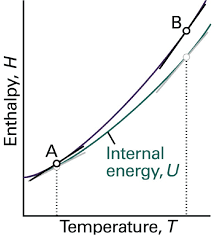To compute for enthalpy at constant pressure, two essential parameters are needed and these parameters are Enthalpy at Point 1 (h1) and Enthalpy at Point 2 (h2).

The formula for calculating enthalpy at constant pressure:

Q = h2 – h1

Where:

Q = Enthalpy at Constant Pressure
h1 = Enthalpy at Point 1
h2 = Enthalpy at Point 2

Given an example;
Find the enthalpy at constant pressure when the enthalpy at point 1 is 20 and the enthalpy at point 2 is 28.

This implies that;

h1 = Enthalpy at Point 1 = 20
h2 = Enthalpy at Point 2 = 28

Q = h2 – h1
Q = 28 – 20
Q = 8

Therefore, the enthalpy at constant pressure is 8 J/Kg.

Calculating the Enthalpy at Point 1 when the Enthalpy at Constant Pressure and the Enthalpy at Point 2 is Given.

h1 = h2 – Q

Where:

h1 = Enthalpy at Point 1
Q = Enthalpy at Constant Pressure
h2 = Enthalpy at Point 2

Let’s solve an example;
Find the enthalpy at point 1 when the enthalpy at constant pressure is 12 and the enthalpy at point 2 is 32.

This implies that;

Q = Enthalpy at Constant Pressure = 12
h2 = Enthalpy at Point 2 = 32

h1 = h2 – Q
h1 = 32 – 12
h1 = 20

Therefore, the enthalpy at point 1 is 20 J/Kg.

## How to Calculate and Solve for Dryness Fraction at Constant Volume Heating or Cooling | Enthalpy

The dryness fraction at constant volume heating or cooling is illustrated by the image below.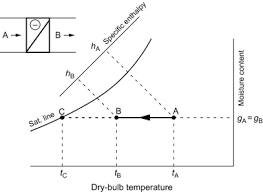To compute for the dryness fraction at constant volume heating or cooling, four essential parameters are needed and these parameters are Specific Volume of Gas at Point 2 (Vg2), Specific Volume of Gas at Point 1 (Vg1), Superheated Steam Temprature (Tsup) and Saturated Temprature (Ts).

The formula for calculating dryness fraction at constant volume heating or cooling:

x = Vg2 Tsup / Vg1 Ts

Where:

x = Dryness Fraction
Vg2 = Specific Volume of Gas at Point 2
Vg1 = Specific Volume of Gas at Point 1
Tsup = Superheated Steam Temprature
Ts = Saturated Temprature

Given an example;
Find the dryness fraction when the specific volume of gas at point 2 is 4, the specific volume of gas at point 1 is 9, the superheated steam temperature is 6 and the saturated temperature is 2.

This implies that;

Vg2 = Specific Volume of Gas at Point 2 =4
Vg1 = Specific Volume of Gas at Point 1 = 9
Tsup = Superheated Steam Temprature = 6
Ts = Saturated Temprature = 2

x = Vg2 Tsup / Vg1 Ts
x = 4 x 6 / 9 x 2
x = 24 / 18
x = 1.33

Therefore, the dryness fraction is 1.33.

Calculating the Specific Volume of Gas at Point 2 when the Dryness Fraction, the Specific Volume of Gas at Point 1, the Superheated Steam Temperature and the Saturated Temperature is Given.

Vg2 = x(Vg1 Ts) / Tsup

Where:

Vg2 = Specific Volume of Gas at Point 2
x = Dryness Fraction
Vg1 = Specific Volume of Gas at Point 1
Tsup = Superheated Steam Temprature
Ts = Saturated Temprature

Let’s solve an example;
Find the specific volume of gas at point 2 when the dryness fraction is 10, the specific volume of gas at point 1 is 4, the superheated steam temperature is 12 and the saturated temperature is 2.

This implies that;

x = Dryness Fraction = 10
Vg1 = Specific Volume of Gas at Point 1 = 4
Tsup = Superheated Steam Temprature = 12
Ts = Saturated Temprature = 2

Vg2 = x(Vg1 Ts) / Tsup
Vg2 = 10(4)(2) / 12
Vg2 = 80 / 12
Vg2 = 6.67

Therefore, the specific volume of gas at point 2 is 6.67

## How to Calculate and Solve for Entropy of Superheated Steam | Enthalpy

The entropy of superheated steam is illustrated by the image below.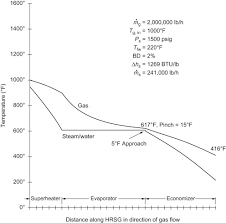To compute for entropy of superheated steam, four essentiala parameters are needed and these parameters are Entropy of Wet Steam (Sg), Constant Pressure (Cps), Superheated Steam Temprature (Tsup) and Saturated Temprature (Ts).

The formula for calculating entropy of superheated steam:

Ssup = Sg + Cps ln(Tsup / Ts)

Where:

Ssup = Entropy of Superheated Steam
Sg = Entropy of Wet Steam
Cps = Constant Pressure
Tsup = Superheated Steam Temprature
Ts = Saturated Temprature

Given an example;
Find the entropy of superheated steam when the entropy of wet steam is 14, the constant pressure is 18, the superheated steam temperature is 10 and the saturated temperature is 12.

This implies that;

Sg = Entropy of Wet Steam = 14
Cps = Constant Pressure = 18
Tsup = Superheated Steam Temprature = 10
Ts = Saturated Temprature = 12

Ssup = Sg + Cps ln(Tsup / Ts)
Ssup = 14 + 18 x ln(10 / 12)
Ssup = 14 + 18 x ln(0.833)
Ssup = 14 + (18 x -0.182)
Ssup = 14 + -3.281
Ssup = 10.71

Therefore, the entropy of superheated steam is 10.71 J/K.

## How to Calculate and Solve for Entropy of Wet Steam | Enthalpy

The entropy of wet steam is illustrated by the image below.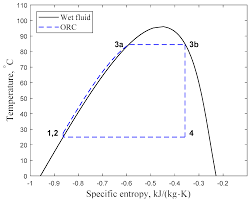To compute for entropy of wet steam, three essential parameters are needed and these parameters are Entropy of Water (Sf), Enthalpy of Vapourisation (hfg) and Saturated Temprature (Ts).

The formula for calculating entropy of wet steam:

Sg = Sf + hfg / Ts

Where:

Sg = Entropy of Wet Steam
Sf = Entropy of Water
hfg = Enthalpy of Vapourisation
Ts = Saturated Temprature

Given an example;
Find the entropy of wet steam when the entropy of water is 12, the enthalpy of vapourisation is 10 and the saturated temperature is 14.

This implies that;

Sf = Entropy of Water = 12
hfg = Enthalpy of Vapourisation = 10
Ts = Saturated Temprature = 14

Sg = Sf + hfg / Ts
Sg = 12 + 10 / 14
Sg = 12 + 0.714
Sg = 12.71

Therefore, the entropy of wet steam is 12.71 J/K.

Calculating the Entropy of Water when the Entropy of Wet Steam, the Entropy of Vapourization and the Saturated Temperature is Given.

Sf = (Sg x Ts) – hfg

Where:

Sf = Entropy of Water
Sg = Entropy of Wet Steam
hfg = Enthalpy of Vapourisation
Ts = Saturated Temprature

Let’s solve an example;
Find the entropy of water when the entropy of wet steam is 10, the enthalpy of vapourization is 5 and the saturated temperature is 2.

This implies that;

Sg = Entropy of Wet Steam = 10
hfg = Enthalpy of Vapourisation = 5
Ts = Saturated Temprature = 2

Sf = (Sg x Ts) – hfg
Sf = (10 x 2) – 5
Sf = 20 – 5
Sf = 15

Therefore, the entropy of water is 15.

## How to Calculate and Solve for Entropy of Evapourisation(Wet Steam) | Enthalpy

The entropy of evapourisation (wet steam) is illustrated by the image below.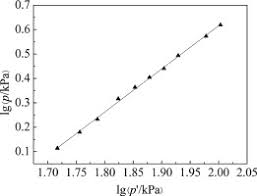To compute for entropy of evapourisation, three essential parameters are needed and these parameters are Enthalpy of Evapourisation (hfg), Saturated Temprature (Ts) and Dryness Fraction (x).

The formula for calculating entropy of evapourisation:

Sevap = xhfg / Ts

Where:

Sevap = Entropy of Evapourisation (Wet Steam)
hfg = Enthalpy of Evapourisation
Ts = Saturated Temprature
x = Dryness Fraction

Given an example;
Find the entropy of evapourisation when the enthalpy of evapourisation is 10, the saturated temperature is 5 and the dryness fraction is 2.

This implies that;

hfg = Enthalpy of Evapourisation = 10
Ts = Saturated Temprature = 5
x = Dryness Fraction = 2

Sevap = xhfg / Ts
Sevap = 2 x 10 / 5
Sevap = 20 / 5
Sevap = 4

Therefore, the entropy of evapourisation is 4 J/K.

Calculating the Enthalpy of Evapourisation when the Entropy of Evapourisation, the Saturated Temperature, the Dryness Fraction is Given.

hfg = Sevap x Ts / x

Where:

hfg = Enthalpy of Evapourisation
Sevap = Entropy of Evapourisation (Wet Steam)
Ts = Saturated Temprature
x = Dryness Fraction

Let’s solve an example;
Find the enthalpy of evapourisation when the entropy of evapourisation is 10, the saturated temperature is 6 and the dryness fraction is 2.

This implies that;

Sevap = Entropy of Evapourisation (Wet Steam) = 10
Ts = Saturated Temprature = 6
x = Dryness Fraction = 2

hfg = Sevap x Ts / x
hfg = 10 x 6 / 2
hfg = 60 / 2
hfg = 30

Therefore, the enthalpy of evapourisation is 30.

## How to Calculate and Solve for Entropy of Evaporation | Enthalpy

The entropy of evaporation is illustrated by the image below.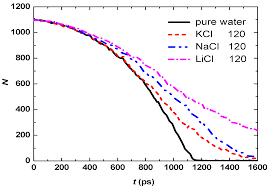To compute for entropy of evaporation, two essential parameters are needed and these parameters are Enthalpy of Evapourisation (hfg) and  Saturated Temprature (Ts).

The formula for calculating entropy of evaporation:

Sevap = hfg / Ts

Where;

Sevap = Entropy of Evapourisation
hfg = Enthalpy of Evapourisation
Ts = Saturated Temprature

Given an example;
Solve for the entropy of evaporation when the enthalpy of evapourisation is 40 and the saturated temperature is 10.

This implies that;

hfg = Enthalpy of Evapourisation = 40
Ts = Saturated Temprature = 10

Sevap = hfg / Ts
Sevap = 40 / 10
Sevap = 4

Therefore, the entropy of evapourisation is 4 J/K.

Calculating the Enthalpy of Evapourisation when the Entropy of Evapourisation and Saturated Temperature is Given.

hfg = Sevap x Ts

Where:

hfg = Enthalpy of Evapourisation
Sevap = Entropy of Evapourisation
Ts = Saturated Temprature

Let’s solve an example;
Find the enthalpy of evapourisation when the entropy of evapourisation is 20 and the saturated temperature is 10.

This implies that;

Sevap = Entropy of Evapourisation = 20
Ts = Saturated Temprature = 10

hfg = Sevap x Ts
hfg = 20 x 10
hfg = 200

Therefore, the enthalpy of evapourisation is 200 J/K.

## How to Calculate and Solve for Entropy of Water | Enthalpy

The entropy of water is illustrated by the image below.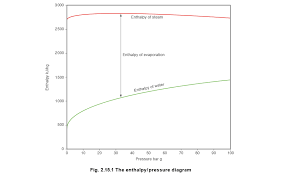To compute for entropy of water, two essential parameters are needed and these parameters are Constant Pressure (Cpw) and Temprature (T).

The formula for calculating entropy of water:

Sp = Cpw ln(T / 273)

Where:

Sp = Entropy of Water
Cpw = Constant Pressure
T = Temprature

Let’s solve an example;
Find the entropy of water when the constant pressure is 40 and the temperature is 10.

This implies that;

Cpw = Constant Pressure = 40
T = Temprature = 10

Sp = Cpw ln(T / 273)
Sp = 40 x ln(10 / 273)
Sp = 40 x ln(0.0366)
Sp = 40 x -3.306
Sp = -132.27

Therefore, the entropy of water is -132.27 J/K.

## How to Calculate and Solve for Internal Energy of Steam (wet steam) | Enthalpy

The internal energy of steam(wet steam) is illustrated by the image below.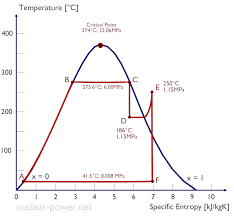To compute for internal energy of steam, five essential parameters are needed and these parameters are Enthalpy of Wet Steam (h), Specific Volume of Gas (vg), Pressure (P), Dryness Fraction (x) and J.

The formula for calculating the internal energy of steam(wet steam):

U = h – Pxvg / J

Where:

U = Internal Energy of Steam(wet Steam)
h = Enthalpy of Wet Steam
vg = Specific Volume of Gas
x = Dryness Fraction
P = Pressure

Given an example;
Find the internal energy of steam (wet steam) when the enthalpy of wet steam is 16, the specific volume of gas is 13, the dryness fraction is 10 and the pressure is 6.

This implies that;

h = Enthalpy of Wet Steam = 16
vg = Specific Volume of Gas = 13
x = Dryness Fraction = 10
P = Pressure = 6

U = h – Pxvg / J
U = 16 – 10 x 6 x 13 / 1
U = 16 – 780 / 1
U = 16 – 780
U = -764

Therefore, the internal energy of steam (wet steam) is -764 J.

Calculating the Enthalpy of Wet Steam when the Internal Energy of Steam, the Specific Volume of Gas, the Dryness Fraction and the Pressure is Given.

h = U + Pxvg / J

Where:

h = Enthalpy of Wet Steam
U = Internal Energy of Steam(wet Steam)
vg = Specific Volume of Gas
x = Dryness Fraction
P = Pressure

Let’s solve an example;
Find the enthalpy of wet steam when the internal energy of steam is 14, the specific volume of gas is 2, the dryness fraction is 6 and the pressure is 3.

This implies that;

U = Internal Energy of Steam(wet Steam) = 14
vg = Specific Volume of Gas = 2
x = Dryness Fraction = 6
P = Pressure = 3

h = U + Pxvg / J
h = 14 + 3(6)(2) / 1
h = 14 + 36 / 1
h = 14 + 36
h = 50

Therefore, the enthalpy of wet steam is 50.

Calculating the Specific Volume of Gas when the Internal Energy of Steam, the Enthalpy of Wet Steam, the Dryness Fraction and the Pressure is Given.

vg = h – U / Px x J

Where:

vg = Specific Volume of Gas
U = Internal Energy of Steam(wet Steam)
h = Enthalpy of Wet Steam
x = Dryness Fraction
P = Pressure

Let’s solve an example;
Find the specific volume of gas when the internal energy of steam is 9, the enthalpy of wet steam is 18, the dryness fraction is 7 and the pressure is 2.

This implies that;

U = Internal Energy of Steam(wet Steam) = 9
h = Enthalpy of Wet Steam = 18
x = Dryness Fraction = 7
P = Pressure = 2

vg = h – U / Px x J
vg = 18 – 9 / 7(2) x 1
vg = 9 / 14 x 1
vg = 0.64 x 1
vg = 0.64

Therefore, the specific volume of gas is 0.64.

## How to Calculate and Solve for Internal Energy of Steam | Enthalpy

The internal energy of steam is illustrated by the image below.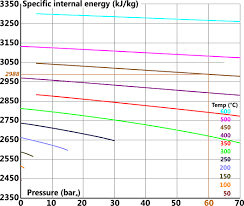To compute for internal energy of steam, four essential parameter are needed and these parameters are Enthalpy of Wet Steam (h), Specific Volume of Gas (vg), Pressure (P) and J.

The formula for calculating internal energy of steam:

U = h – Pvg / J

Where:

U = Internal Energy of Steam
h = Enthalpy of Wet Steam
vg = Specific Volume of Gas
P = Pressure

Given an example;
Find the internal energy of steam when the enthalpy of wet steam is 20, the specific volume of gas is 14 and the pressure is 8.

This implies that;

h = Enthalpy of Wet Steam = 20
vg = Specific Volume of Gas = 14
P = Pressure = 8

U = h – Pvg / J
U = 20 – 8 x 14 / 1
U = 20 – 112 / 1
U = 20 – 112
U = -92

Therefore, the internal energy of steam is -92J.

Calculating the Enthalpy of Wet Steam when the Internal Energy of Steam, the Specific Volume of Gas and the Pressure is Given.

h = U + Pvg / J

Where:

h = Enthalpy of Wet Steam
U = Internal Energy of Steam
vg = Specific Volume of Gas
P = Pressure

Let’s solve an example;
Given that the internal energy of steam is 28, the specific volume of gas is 12 and the pressure is 8. Find the Enthalpy of wet steam?

This implies that;

U = Internal Energy of Steam = 28
vg = Specific Volume of Gas = 12
P = Pressure = 8

h = U + Pvg / J
h = 28 + 8(12) / 1
h = 28 + 96
h = 124

Therefore, the enthalpy of wet steam is 124J/Kg.